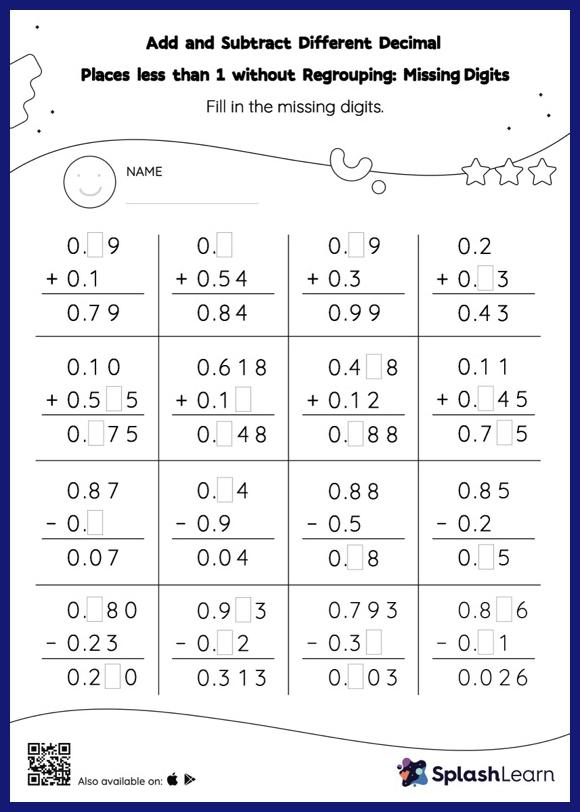# Add and Subtract Different Decimal Places less than 1 without Regrouping: Missing Digits Worksheet

Home > Add and Subtract Different Decimal Places less than 1 without Regrouping: Missing DigitsDoes your child know how to add and subtract different decimal places less than 1 without regrouping? Invite them to practice this concept here. While adding and subtracting decimals, students use the relationship between addition and subtraction to find the missing number. They do not need to regroup numbers in add and subtract different decimal places less than 1 without regrouping worksheet. In this worksheet, students practice solving problems using the column method. This method is especially helpful with problems involving bigger multi-digit numbers as the format provides an easy structure to follow.